# Reproducing Leslie N. Smith's papers using fastai¶

The following papers by Leslie N. Smith are covered in this notebook :-

1. A disciplined approach to neural network hyper-parameters: Part 1 -- learning rate, batch size, momentum, and weight decay. paper
2. Super-Convergence: Very Fast Training of Neural Networks Using Learning Rates. paper
3. Exploring loss function topology with cyclical learning rates. paper
4. Cyclical Learning Rates for Training Neural Networks. paper

Although, the main aim is to reproduce the papers but a lot of research has been done since than and thus where needed I would change some things to match the state of the art practices. Most of these things are taught in the fastai courses, namely Practical Deep Learning for Coders, v3.

If you are not familiar with fastai, it is a deep learning library build on top of PyTorch and it contains the implementations of most of the state of the art practices, which keep changing over time. As a result of this you can get state of the art results in most of the tasks by using the defaults of the library.

How this notebook is structured. I would explain all the concepts discussed in the paper and would provide a walkthrough with a CIFAR-100 example along the way. So there would be explanation of the topic and then the code for that. If you are to use these techniques for your own work, you can follow along the notebook from top-to-bottom. For the implementations of some concepts, I would use the fastai built in functions as fastai provides a callback system that really helps a lot when working on projects in real life. So if you do not know fastai, you can watch the course mentioned above or read the docs, as the docs contains ample examples. For Tensorflow users, as of now I am not aware if some library provides this much functionality as fastai but you can still follow on as the concepts discussed are general, only the implementation is different.

If you are learning fastai, this notebook can be a very good tutorial on how to use the vision API in fastai.

1. Summary of hyper-parameters
2. Hyper-params not discussed
3. Things to remember
4. Underfitting vs Overfitting
5. Deep Dive into Underfitting and Overfitting
1. Underfitting
2. Overfitting
6. Choosing Learning Rate
1. Cyclic Learning Rate (CLR) and Learning Rate Test
2. ResNet-56
3. Cyclic Learning Rate
4. Difference from Original paper
5. One-cycle policy summary
6. Learning rate finder test
7. Introducing Super-Convergence
1. Testing Linear Interpolation tests
2. How it was found in the first place?
3. Coding Linear INterpolation
8. Explanation behind Super-Convergence
9. Choosing Momentum
1. Some good values of momentum to test
10. Choosing Wight Decay
1. How to set the value
11. Train a final classifier model with above param values
In [ ]:
# fastai imports most of the things that you need like numpy
# and much more, so no need to import them again in our work.
from fastai import *
from fastai.vision import *


Although deep learning has produced dazzling successes for applications of image, speech and video processing in the past few years, most trainings are done with suboptimal hyper-parameters, requiring unnecessarily long training times. Setting the hyperparameters reamains a black art that requires years of experience to acquire. So I present several efficient ways to set the hyper-parameters that significantly reduce the training time and improves performance. Specifically, we examine the training and validation/test loss for subtle clues of underfitting and overfitting and suggest guidelines for moving toward the optimal balance point.

Note: Should I use hyper-parameter tuning library? I asked this question on the fastai forums and Jeremy replied, "I would suggest avoiding black-box hyperparam tuning wherever possible. I've only used it once in my life, and even then it wasen't really a win. Instead, use the techniqeus we've learned to try carefully selected choices."

And I agree with that. Most of the times there is not much need to use these libraries and as we would soon find that most of the hyper-parameters are linked with each other, so we should tune them accordingly. An argument can be made for Bayesian Optimizations, but I have not used them and I find the techniques discussed here much simpler and safer.

## Summary of hyper-parameters¶

• Learning rate :- Use learning rate finder test and get the maximum value of learning rate that you would use in the 1cycle policy.
• Batch size :- The largest value that fits on your GPU. You can use batch sizes like 20 that are not powers of two. The performance drop that is discussed when using batch size that are not powers of 2 is true, but we can ignore it if we want.
• Momentum :- Use cyclic momentum in most of the tasks. When you are using GANs use a constant value of momentum.
• Weight Decay :- Larger value when using smaller dataset and model. Smaller value when using bigger datasets and models. Use a constant value.

### Hyper-params not discussed¶

• Architecture
• Regularization
• Dataset

## Things to remember¶

1. Setting hyperparameters is very important. Every dataset would have their own set of hyperparameter values, and setting the right hyper-parameter values should be your only priority I would say initially.
2. Regularization vs weight decay. In regularization we subtract something from the loss function, while in weight decay we subtract something from the parameter update step.
3. When we use modern architectures like Resnet it is better to use weight decay than L2 regularization.
4. You can set all your hyper-parameters in a few epochs.

## Underfitting vs Overfitting¶

The basis of this notebook is based on the concept of underfitting vsersus overfitting. Specifically, it consists of examining the training's test/validation loss for clues of underfitting and overfitting in order to strive for the optimal set of hyper-parameters. By observing and understanding the clues available early during training, we can tune our architecture and hyper-parameters with short runs of a few epochs. In particular, by monitoring validation/test loss early in the training, enough information is available to tune the architecture and hyper-parameters and this eliminates the necessity of running complete grid or random searches.

One key finding in the paper is that the total regularization needs to be in balance for a given dataset and architecture. And it was found that learning rate, momentum and regularization are tightly coupled and optimal values must be determined together. This means that if you set a learning rate as a large value than other regularizations like momentum must come down, so that the total remains preserved.Fig 1: The figure shows the optimal capacity that falls between underfitting and underfittingFig2(a) See the top-left corner. You can see a similar bump as was shown in Fig1. This is an early sign of overfitting. Fig2(b)The overfitting can be see around 3500 where our valid loss starts becoming worse

Fig 2: Figure showing on how you can detect early signs of overfitting when working on your data

An important thing to see here is in some cases like that shown in Fig2(a) where you see that early overfitting but after that your test loss still decreases a little. This can be misleading in some cases where one can get blindsided by reduction in the amoung of test loss. So draw your losses and see whether you overfit or not. You should not use a model that overfit early as after that point you can consider your model just learning some examples to reduce test loss, which results in poor generalization.

## Deep Dive into Underfitting and Overfitting¶

### Underfitting¶

Underfitting is when the machine learning model is unable to reduce the error for either the test or training set, which is due to the under capacity of the machine learning model i.e. it is now pwerful enough to fit the underlying complexities of the data distributions. Whenever your valid loss is less than the training loss than it means your model is underfitting.Fig 3: Underfitting is characterized by a continously decreasing test loss, rather than a horizontal plateau.

### Overfitting¶

Overfitting is when your model is too powerful, such that the model starts fitting the training set too well. Using small learning rates can exhibit some overfitting behaviour (as shown in Fig2(a))Fig4(a): When using WD=1e-4, the loss reaches a minimum near lr=0.002, then begins to increase displaying overfitting. For WD=4e-3, the loss is stable over a large range of values and attains a lower loss value, indicating that the later WD value is superior to the former Fig4(b): While the blue curve shows underfiting as the loss keeps decreasing while the red curve shows overfiting where the loss first decreased and then increased.

Fig 4: Increasing validation/test loss indicates overfitting. In fig (a) the sharp increase in loss for yellow curve near lr=0.005 is not a sign of overfitting but is caused by instabilities in the training due to high learning rate.

## Choosing Learning Rate¶

If the learning rate is too small overfitting can occur. Large learning rates help to regularize the training but if the learning rate is too large, the training will diverge. Hence a grid search of short runs to find learning rates that converge or diverge is possible but there is an easier way.

By training with high learning rates we can reach a model that gets 93% accuracy in 70 epochs which is less than 7k iterations (as opposed to the 6rk iterations which made roughly 360 epochs in the original paper of Resnet).

### Cyclical Learning Rates(CLR) and learning rate test¶

As a motivation for using this approach see the below figure, just how fast we can get the state of the art results is shown. The reasoning for this is discussed in the next topic.Sylvain Gugger has written two very good blog posts explaining this topic, so I would recommend you to read those first.

• How Do You Find A Good Learning Rate link

Here I just summarize the topic with important details, for a detailed overview refer to the above two links.

In [ ]:
# Load CIFAR-100 data. To downlaod the data refer to this link
# https://course.fast.ai/datasets.

# 'Path' is a python package which makes working with directory
# names a lot easier, to import it use from pathlib import Path
path = Path('/home/kushaj/Desktop/Data/cifar100/')
path.ls()

Out[ ]:
[PosixPath('/home/kushaj/Desktop/Data/cifar100/test'),
PosixPath('/home/kushaj/Desktop/Data/cifar100/models'),
PosixPath('/home/kushaj/Desktop/Data/cifar100/train')]
In [ ]:
# Both test and train folder contains images in the Imagenet format
# where every image is placed in a folder with the same name as the
# class of the image.

In [ ]:
# Create a databunch
src = (ImageList.from_folder(path)
.split_by_folder(valid='test')
.label_from_folder())

In [ ]:
data = (src.transform(get_transforms(), size=(32,32))
.databunch(bs=256, val_bs=512, num_workers=8)
.normalize(cifar_stats))

cifar_stats

Out[ ]:
([0.491, 0.482, 0.447], [0.247, 0.243, 0.261])
In [ ]:
data.show_batch(rows=3, figsize=(4,4))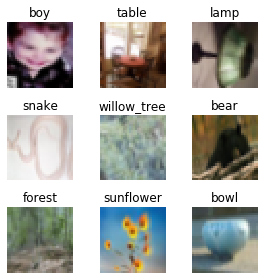After creating the databunch we have done the following things

• Added data augmentation with the following transforms and the size of images is taken as (32,32)
• Normalied the images with the CIFAR_STATS
• I am using a batch size of 256 for both trian and valid sets

### Resnet-56¶

The reason I am not using the original resnet models is due the two downsampling layers in the starting of the resnet model, namely conv and max_pool. If we use these layers than our image size is reduced to 8x8 after just two layers.

So Resnet-56 was also used in the orginal papers and in genral it is a good model for small size images.

In [ ]:
# Extra Classes for the head of our model
"Layer that concats AdaptiveAvgPool2d and AdaptiveMaxPool2d."
def __init__(self, sz=None):
"Output will be 2*sz or 2 if sz is None"
super().__init__()
self.output_size = sz or 1
def forward(self, x):

class Flatten(nn.Module):
"Flatten x to a single dimension, often used at the end of a model. full for rank-1 tensor"
def __init__(self, full:bool=False):
super().__init__()
self.full = full
def forward(self, x):
return x.view(-1) if self.full else x.view(x.size(0), -1)

class BasicBlock(nn.Module):
def __init__(self, c_in, c_out, stride=1):
super().__init__()
self.conv1 = nn.Conv2d(c_in, c_out, kernel_size=3, stride=stride, padding=1, bias=False)
self.bn1 = nn.BatchNorm2d(c_out)
self.conv2 = nn.Conv2d(c_out, c_out, kernel_size=3, stride=1, padding=1, bias=False)
self.bn2 = nn.BatchNorm2d(c_out)

if stride != 1 or c_in != c_out:
self.shortcut = nn.Sequential(
nn.Conv2d(c_in, c_out, kernel_size=1, stride=stride, bias=False),
nn.BatchNorm2d(c_out)
)

def forward(self, x):
shortcut = self.shortcut(x) if hasattr(self, 'shortcut') else x
out = F.relu(self.bn1(self.conv1(x)))
out = self.bn2(self.conv2(out))
out += shortcut
return F.relu(out)

class Resnet(nn.Module):
def __init__(self, num_blocks=[9,9,9], num_classes=100):
super().__init__()
self.conv1 = nn.Conv2d(3, 16, kernel_size=3, stride=1, padding=1, bias=False)
self.bn1 = nn.BatchNorm2d(16)
self.layer1 = self.make_group(16, 16, num_blocks, stride=1)
self.layer2 = self.make_group(16, 32, num_blocks, stride=2)
self.layer3 = self.make_group(32, 64, num_blocks, stride=2)

Flatten(),
nn.BatchNorm1d(128),
nn.Dropout(0.25),
nn.Linear(128, 128, bias=True),
nn.ReLU(inplace=True),
nn.BatchNorm1d(128),
nn.Dropout(0.5),
nn.Linear(128, num_classes, bias=True)
)

def make_group(self, c_in, c_out, num_blocks, stride):
layers = [BasicBlock(c_in, c_out, stride)]
for i in range(num_blocks-1):
layers.append(BasicBlock(c_out, c_out, stride=1))
return nn.Sequential(*layers)

def forward(self, x):
out = F.relu(self.bn1(self.conv1(x)))
out = self.layer1(out)
out = self.layer2(out)
out = self.layer3(out)
return out

In [ ]:
learn = Learner(data, Resnet(), metrics=[accuracy, top_k_accuracy], callback_fns=[ShowGraph])


Till now we have done the following:

• Fot out data, which is stored in data as a databunch
• A Resnet model is created
• A learner object is created which is names learn
• AdamW is used as the optimization function
• CrossEntropyLoss is used as loss function

### Cyclic Learning Rate¶

The essence of this learning rate policy comes from the observation that increasing the learning rate might have a short term negative effect and yet achieve a longer term beneficial. So we vary our learning rate from a small value to large value than back to small value. This is termed as one complete cycle.This learning rate policy is taken from the original paper, but that was few years back. Now we use a cosine policy that lookes something like this.

In [ ]:
# Skip the code for now
learn.fit_one_cycle(1, max_lr=1e-2)
learn.recorder.plot_lr()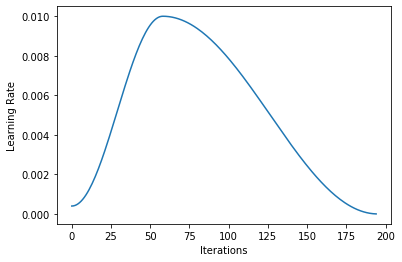If you have any question on why is the learning rate policy defined as this or in general to the learning rate policy in fastai, I have answered all the questions in the forums which you can check here. There I clearly explain why we use a learning rate policy that looks like the one shown above and what are the reasons behind choosing the deafults. (The main reason is we want to train our model at higher learning rates and then fine-tune them at lower learning rates).

For the implementation of this, you can check this where I implement the one-cycle policy in pure pytorch.

### Difference from original paper¶

There are some changes that you would notice from the original paper. First, in the papers for most of the cases accuracy is used as the metric for most of the graphs, while I use loss values. The reason loss values are used to compare hyper-parameter value is loss is the actual thing that is being optimized we want to reduce it the most.

### One-cycle policy summary¶

There are two phases, first learning rate increases from small lr to maximum value and in the second phase it decreased from the maximum value to the minimum value (the minimum value is smaller than the starting value in the first phase). In implementation, you only need to define the maximum value of the learning rate and the minimum values would be calculated appropriately.

### Learning rate finder test¶

In order to find the maximum value of leanring rate (max_lr) we using a learning rate test. In this test we start with a small value of learning rate (1e-7) and then increase this value to a maximum of 10 as shown in the figure below.

In [ ]:
learn.lr_find()
learn.recorder.plot_lr()

LR Finder is complete, type {learner_name}.recorder.plot() to see the graph.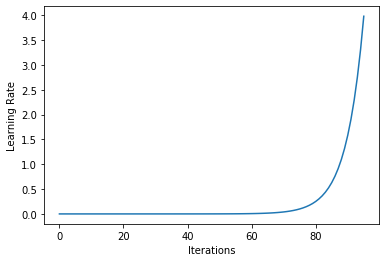In fastai this test can be done using learn.lr_find. After running this test we plot a diagram of the loss values for the different values of learning rate and try to find the maximum value of learning rate that we can use.

In [ ]:
learn.lr_find(start_lr=1e-7, end_lr=10, num_it=100)

LR Finder is complete, type {learner_name}.recorder.plot() to see the graph.

In [ ]:
# We plot the loss vs learning rate
learn.recorder.plot()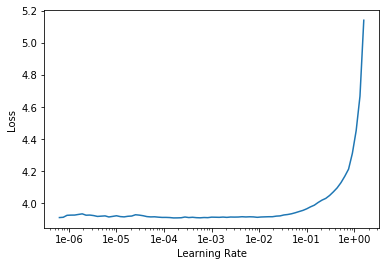Now you need to look at the above figure and select the lr value that you want to use. I would use lr=1e-2. The art of selecting good values comes down to practice, just try the values you are confused about and select the one that gives the best results. There are certain guidelines that you should follow while finding the lr value

• Select the one where the loss is small for larger amount of time
• When loss begins to increase, select a value that is 10 times less

For experimentation, let us see what the resutls are when I use max_lr=1e-2 and max_lr=1e-1

In [ ]:
# In fastai there fit_one_cycle function that trains your model using the 1cycle policy
# So you don't have to code up anything
learn = Learner(data, Resnet(), metrics=[accuracy, top_k_accuracy], callback_fns=[ShowGraph])
learn.fit_one_cycle(3, max_lr=1e-2)

epoch train_loss valid_loss accuracy top_k_accuracy time
0 4.117561 4.697643 0.047400 0.175600 01:48
1 3.663519 3.413377 0.173200 0.446900 01:47
2 3.335882 3.095275 0.239100 0.529900 01:48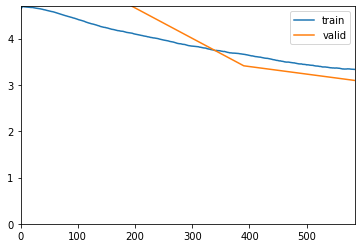In [ ]:
learn = Learner(data, Resnet(), metrics=[accuracy, top_k_accuracy], callback_fns=[ShowGraph])
learn.fit_one_cycle(3, max_lr=1e-1)

epoch train_loss valid_loss accuracy top_k_accuracy time
0 4.334694 4.820587 0.036000 0.156500 01:51
1 3.994016 3.712073 0.109600 0.351700 01:52
2 3.565970 3.270659 0.197700 0.490200 01:46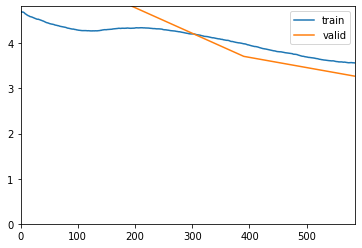As you can see clearly max_lr=1e-2 is better than max_lr=1e-1. Athough this test took around 11 minutes but we easily got the value of lr that we should use. With enough practice you can predict the best value of max_lr from directly the lr_find graph but whenever you are confused running some epochs can help.

## Introducing Superconvergence¶

Now we know what is 1cycle policy and how we can use it for our own work in fastai, next I want to discuss Superconvergence which is basically what we are getting using the 1cycle policy.Test accuracy for learning rate range = 0.001 - 1 for ResNet-52, ResNet 56 and ResNet 60

There are two noteworthy things to see in this figure. First is the dip in the accuracy around LR=0.1. Second is the consistently high test accuracy over a large span of learning rates (LR=0.25 to 1.0), which is unusual. Seeing these unsual facts, experiments were carried out using cyclic learning rate and the following counterintuitive things results appeared.(a) Cyclical learning rate between LR=0.1 and LR=0.35 with stepsize=10K (b) Super-convergence with CLR = 0.1-1.0 versus standard training with initial LR=0.35

Fig: Test accuracies for Resnet-56 on CIFAR-10. Note the log scale used for the vertical axis.

You can see an anomly that occurs as the LR increases from 0.1 to 0.35. The training loss increases sharply by four orders of magnitude at a learning rate of approximately 0.255 but training convergence resumes at larger learning rates. In addition, there are divergenct behaviours between test accuracy and loss curves that are not easily explained.In the first cycle, when the learning rate is increasing from 0.13 to 0.18 the test loss increases but the test accuracy also increases. This simultaneous increase in the test loss and the test accuracy also occurs in the second cycle as the learning rate decrease from 0.35 to 0.1 and in various portions of subsequent cycles.

Another interesting fact can be see from the second figure. The cyclic learning rate method is able to get 0.93 accuracy after just once cycle and it remains the same for the subsequent cycles, while the standard approach of using a constant learning rate manages to achieve the accuracy close to 0.93 at about 5 times more iterations.

### Superconvergence¶

Super-convergence refers to this phenomenon where a network is trained to a better final test accuracy compared to tradional training, but with fewer iterations and a much larger learning rate.

### Testing linear interpolation results¶

In the Exploring Loss Function Topology with Cyclical Learning Rates (only 3 pages) an important topic of networkinterpolation is discussed which shows that the solution found by each cycle is different from each other (intuitively you can think the solutions as belonging to different valleys). So we can interpolate solutions from different cycles and we can except better accuracy. So I test this fact now?

Interpolation between two values is defined as### How it was found in the first place?¶

Before getting into code, I would discuss how this fact was found. So interpolations between different cycle weights was tested and the plots for interpolation were drawn for the train and test loss. One of the figure is shown below(a) Training and test loss for interpolation between two network states from regular ResNet training. If both the values are for the same minima than this is what we would get, two concave shapes. Training and test loss for interpolation between two CLR solutions. If there is a peak between two solutions then the two minimas are different solutions.
Fig: Results of network interpolation for differently trained models

From this plot it was observed that the solution found by each cycle indeed belonged to different minima. Also an addional noteworthy feature, some amount of regularization is possible through interpolating between two solutions. The minima for training loss are at a=0.0 and 1.0 but the test loss minima are slightly offset towards the center.

### Coding Linear Interpolation¶

To check the results for the above topic the following things would be done:

1. Do some training epochs on the training dataset and get the weights for diferent cycles
2. Make a plot of interpolation between different weight values

To modify our training loop callbacks would be used. I consifer callbacks to tbe the most important feature of fastai as it allows infinite customization without chaning the training loop.

In [ ]:
class GetWeights(Callback):
def __init__(self, learn, save_list=[5, 7]):
self.learn = learn
self.save_list = save_list

def on_train_begin(self, **kwargs):
self.weights = {}

def on_epoch_end(self, **kwargs):
if kwargs['epoch'] in self.save_list:
weights = learn.model.state_dict()
for k, v in weights.items():
weights[k] = v.cpu()
self.weights[kwargs['epoch']] = weights

def get_weights(self):
return self.weights

learn = Learner(data, Resnet(), metrics=[accuracy, top_k_accuracy], callback_fns=ShowGraph)
getWeights = GetWeights(learn)

In [ ]:
learn.fit_one_cycle(8, max_lr=1e-2, callbacks=getWeights)

epoch train_loss valid_loss accuracy top_k_accuracy time
0 4.191520 4.533091 0.053900 0.195400 01:54
1 3.791083 4.001433 0.102000 0.318300 01:53
2 3.429356 3.574863 0.161400 0.429000 01:50
3 3.125475 3.035374 0.231600 0.533600 01:53
4 2.832539 2.619795 0.312300 0.643800 01:53
5 2.584690 2.346036 0.374700 0.704900 01:53
6 2.407087 2.109318 0.422400 0.756300 01:53
7 2.304121 2.082097 0.429800 0.761200 01:48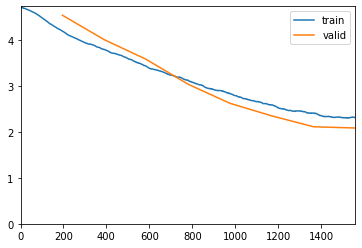In [ ]:
weights = getWeights.get_weights()
w1 = weights
w2 = weights

In [ ]:
# Just to verify that it worked. The loss value is same as above so it worked
learn.validate()

Out[ ]:
[2.3460364, tensor(0.3747), tensor(0.7049)]
In [ ]:
# Do interpolation
def interpolate(alpha):
w_new = {}
keys = list(w1.keys())
for key in keys:
w_new[key] = alpha*w1[key] + (1 - alpha)*w2[key]
return w_new

In [ ]:
alpha_range = np.linspace(start=-0.5, stop=1.5, num=100)
train_loss = []
val_loss = []
for i, alpha in enumerate(alpha_range):
print(f'{i}/{len(alpha_range)} started')
w_new = interpolate(alpha)
loss1, _, _ = learn.validate()
loss2, _, _ = learn.validate(data.train_dl)

val_loss.append(loss1)
train_loss.append(loss2)

In [ ]:
plt.figure(figsize=(10,6))
plt.plot(alpha_range, train_loss, 'b')
plt.plot(alpha_range, val_loss, 'r')
plt.show()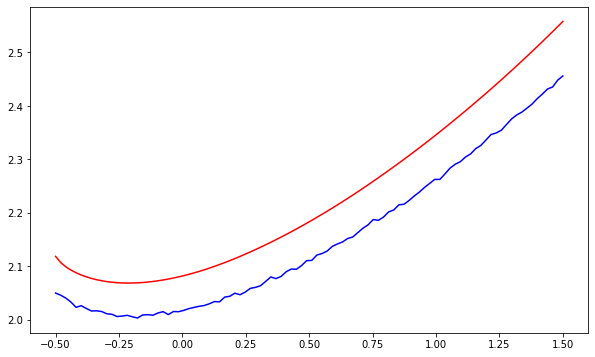In [ ]:
alpha_range = np.linspace(start=-10, stop=4, num=50)
train_loss2 = []
val_loss2 = []
for i, alpha in enumerate(alpha_range):
print(f'{i}/{len(alpha_range)} started')
w_new = interpolate(alpha)
loss1, _, _ = learn.validate()
loss2, _, _ = learn.validate(data.train_dl)

val_loss2.append(loss1)
train_loss2.append(loss2)

In [ ]:
plt.figure(figsize=(10,6))
plt.plot(alpha_range, train_loss2, 'b')
plt.plot(alpha_range, val_loss2, 'r')
plt.show()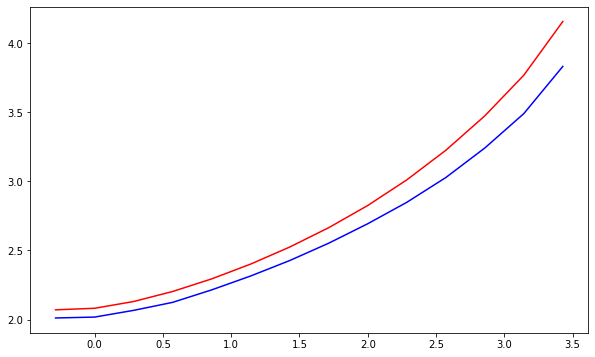Losses went nan for the initial values. Seeing this we cannot combine both of them, but the reason being in the paper SGD was used while here we are using AdamW with cyclic momentum by default so it changes up the situation by a lot. But it is a fun experiment.

## Explanation behind Super-Convergence¶

As we discussed earlier, one of the indicators of super-convergence are the consistent high value of accuracies with increasing learning rate. Cyclic Learning which allowed super-convergence is indeed a combination of Curriculm learning and simulated annealing. Also, as the amount of data decreases the gap in performance between the result of standard training and super-convergence increases. Specifically, with a peicewise constant learning rate schedule the training encounters difficulties and diverges along the way.(a) Visualization of how training traverses a loss function topology (b) A close up of the end of training for figure (a)
The 3-D visualizations from Goodfellow et al. . The z-axis represents the loss potential.

The above figure gives an intuitive understanding of how super-convergence happens. The blue line in the figure represents the trajectory of the training while converging and the x's indicate the location of the solution at each iteration and indicates the progress made during the training.

The while loss surface can be divided into 3 phases:-

1. In early training, the learning rate must be small in order for the training to make progress in appropriate direction. As you can see in the figure a significant progress is made in those early iterations (the part where we descend the valley)
2. Now as the slope decreases so does the amount of progress made per iteration and little improvement occurs over the bulk of the iteration. This is the reason why we increase the learning rate to high values, so that we can quickly move over this region.
3. As we approach the bottom of the loss surface (you can think of it as bottom of valley with bumps), so here we need to slow down and get to the bottom of these bumps i.e. why we decrease the value of learning rate to minimum value so that we can fine-tune our final result.

### A quick summary¶

Initially when the learning starts there is huge slope and we move down it quickly, now there is a straight out path and we make very less progress through each iterations. At the end we enter a valley and we have to move towards the minima. Cyclic Learning Rate solves it. We start with small learning rate to get over that initial big slope. Then we increase the learning rate to quickly move through the straight path and then we again decrease learning rate to move through the valley.

## Choosing momentum value¶

Choosing the value of momentum depends on the task at hand. So therea are two options either use cyclic momentum or constant value of momentum. I would directly give you the results here

• IF you are training GAN's or any task where you are quickly shifting between different models (like in GANs we shift between generator and discriminator) you should use constant value of momentum. Because you will not have enough time to get the benefits of cyclic momentum in these tasks.
• Cyclic momentum should be your default choice for other tasks. And for the momentum values they should be high->low i.e. opposite of learning rate.

In implementation we would specify the minimum and maximum value of momentum and move from max->min and then min->max. Below I show the cyclic learning rate and cyclic momentum values side by side.

In [ ]:
learn = Learner(data, Resnet())
learn.fit_one_cycle(1)
learn.recorder.plot_lr(show_moms=True)

epoch train_loss valid_loss time
0 4.013027 3.831154 01:47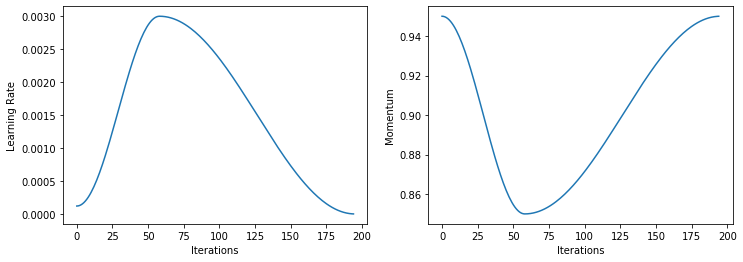The reason behind using this approach is we have to keep the total regularization in check. So if I use higher learning rates which provide regularization on their own, I do not need high values of momentum (as high momentum value would also provide large regularization values) and this would ensure that this results in convergence for a longer range of learning rate and faster convergence. The optimal learning rate is dependent on the momentum and momentum is dependent on the learning rate.

### Some good values of momentum to test¶

This is a difficult question to answer. Genrally the default of (0.95 - 0.85) works good, but there are some values that you can test

• 0.99 - 0.90
• 0.95 - 0.85
• 0.99
• 0.9
• 0.85

Note:- In practice, we would choose this value in combination with the value of weight decay. But for quick demonstration I show how to choose value of momentum only.

I test for the first two cases.

In [ ]:
learn = Learner(data, Resnet(), metrics=[accuracy, top_k_accuracy], callback_fns=ShowGraph)
learn.fit_one_cycle(3, max_lr=1e-2, moms=(0.99, 0.90))

epoch train_loss valid_loss accuracy top_k_accuracy time
0 4.074203 4.473627 0.058200 0.209500 01:45
1 3.603846 3.422370 0.168400 0.436200 01:46
2 3.306487 3.084147 0.243600 0.533900 01:47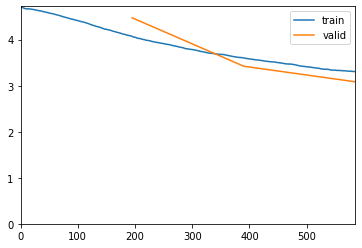In [ ]:
learn = Learner(data, Resnet(), metrics=[accuracy, top_k_accuracy], callback_fns=ShowGraph)
learn.fit_one_cycle(3, max_lr=1e-2, moms=(0.95, 0.85))

epoch train_loss valid_loss accuracy top_k_accuracy time
0 4.091744 4.376647 0.064800 0.228900 01:47
1 3.645397 3.546236 0.142300 0.401900 01:47
2 3.348282 3.130295 0.232100 0.524700 01:46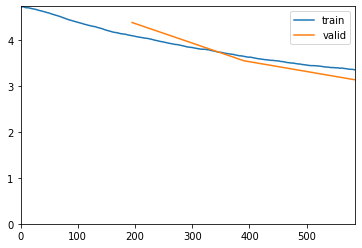The testing took around 11 minutes and I think the value of 0.99-0.90 for momentum is better than 0.95-0.85. This is it. Using this method you can get creative and test out many of your hyperparameter choices without using any external libraries which in most cases do not even work.

One of the things that you may be wondering what to do if I have large datasets like Imagenet dataset where every epoch takes hours to run. In that situation I would suggest to take a small smaple of the dataset and adjust your hyper-parameters using those. Generally you don't need to have very large validation set, a subset of the validation set can also work provided that subset is a good representation of the actual validation set. You can become creative when using large datasets where for the training dataset you treat a specific number of batches as a single epoch. For example, if my dataset can be divided into 100 batches and I decide every 20 batches would be treated as a single epoch. So after 100 batches I would have done 5 epochs instead of 1.

Note:- I did not even need 3 epochs to decide which value is better. If we just compare the resutls from the first two epochs we can clearly make our choice without having to do third epoch.

## Choosing Weight Decay¶

Weight decay is not like momentum and learning rate and the best value should remain constant throughout the training. Since the networks performance is dependent on a proper weight decay value, a grid search is worthwhile and differences are visible early in the training. That is the validation loss early is sufficient for determining a good value.

So to set the value of weight decay you should run combined runs using different values of weight decay and momentum and possibly learning rate. Generally what I found good is using the lr_range test to get the value of learning rate and then adjust momentum and weight decay accordingly using various combinations.

The reason we can avoid finding the values of learning rate, momentum and weight decay simultaneously is all of these hyper-parameters are coupled and if we set a low value for some param we can set higher values of other params, so as to keep the total regularization in check.

### How to set the value¶

This requires a grid search to determine the proper magnitude but usually does not require more than one significant figure accuracy. Use your knowledge of the dataset and architecture to decide which values to use. For example, a more complex dataset requires less regularization so test smaller weight decay values such as 1e-4, 1e-5, 1e-6. A shallow architecture requires more regularization so test larger weight decay values such as 1e-2, 1e-3, 1e-4. The reason being complex datasets provide regularization on their own and other regularizations should be reduced.

So if you guess that 1e-4 should be a good value than test 3e-5, 1e-4, 3e-4. How I chose the value 3? So if you think your weight decay best value lies between 10-4 and 10-3, than you should choose the value 10-3.5 i.e take average of the exponent. You can keep going in this way.

To make testing simpler I would use cyclic momentum as (0.99-0.90) and find the optimal value of weight decay. The reason I do not need to change the momentum value here is that momentum is already changing from 0.99 to 0.9 as we are using cyclic momentum, so in implementation we can first find a good cyclic momentum value and then test out weight decay values.

In [ ]:
# WD = 0
learn = Learner(data, Resnet(), metrics=[accuracy, top_k_accuracy])
learn.fit_one_cycle(2, max_lr=1e-2, moms=(0.99-0.90), wd=0)

epoch train_loss valid_loss accuracy top_k_accuracy time
0 4.114937 4.064409 0.061100 0.235800 01:56
1 3.662782 3.437547 0.168800 0.436900 01:46
In [ ]:
# WD = 1e-4
learn = Learner(data, Resnet(), metrics=[accuracy, top_k_accuracy])
learn.fit_one_cycle(2, max_lr=1e-2, moms=(0.99-0.90), wd=1e-4)

epoch train_loss valid_loss accuracy top_k_accuracy time
0 4.124258 3.914982 0.085900 0.281900 01:48
1 3.668026 3.459805 0.171700 0.436300 01:47
In [ ]:
# WD = 1e-5
learn = Learner(data, Resnet(), metrics=[accuracy, top_k_accuracy])
learn.fit_one_cycle(2, max_lr=1e-2, moms=(0.99-0.90), wd=1e-5)

epoch train_loss valid_loss accuracy top_k_accuracy time
0 4.139386 4.032632 0.074400 0.247900 01:49
1 3.692349 3.504380 0.165300 0.419900 01:49
In [ ]:
# WD = 1e-3
learn = Learner(data, Resnet(), metrics=[accuracy, top_k_accuracy])
learn.fit_one_cycle(2, max_lr=1e-2, moms=(0.99-0.90), wd=1e-3)

epoch train_loss valid_loss accuracy top_k_accuracy time
0 4.130610 4.117643 0.064100 0.235600 01:49
1 3.725400 3.531017 0.156800 0.414100 01:48

From the above results is clear that WD=1e-4 is the best. So now I test around 1e-4 with the same rule as explained above. So I take the average of -4 and -3 and I get -3.5, so the multiplier I choose is 10-3.5 which is approx 3.12*10-3. The values I test are 3x10-4 and 3x10-5

In [ ]:
learn = Learner(data, Resnet(), metrics=[accuracy, top_k_accuracy])
learn.fit_one_cycle(2, max_lr=1e-2, moms=(0.99-0.90), wd=3e-4)

epoch train_loss valid_loss accuracy top_k_accuracy time
0 4.128520 4.023188 0.069500 0.250500 01:45
1 3.689037 3.464480 0.162000 0.426000 01:46
In [ ]:
learn = Learner(data, Resnet(), metrics=[accuracy, top_k_accuracy])
learn.fit_one_cycle(2, max_lr=1e-2, moms=(0.99-0.90), wd=3e-5)

epoch train_loss valid_loss accuracy top_k_accuracy time
0 4.116028 3.965793 0.077100 0.269000 01:46
1 3.712324 3.490986 0.166200 0.425000 01:46

The final weight decay value that I choose after seeing the above resuls is 1e-4. In this way you can test different hyperparameter values and see which performs the best.

## Train a final classifier model with the above param values¶

In [ ]:
learn = Learner(data, Resnet(), metrics=[accuracy, top_k_accuracy], callback_fns=ShowGraph)

In [ ]:
learn.fit_one_cycle(15, max_lr=1e-2, moms=(0.99-0.9), wd=1e-4)

epoch train_loss valid_loss accuracy top_k_accuracy time
0 4.271368 4.100077 0.058000 0.230000 01:47
1 3.935396 4.278841 0.058900 0.215700 01:46
2 3.700406 3.707660 0.128600 0.364100 01:46
3 3.440132 3.624694 0.159000 0.405800 01:46
4 3.107491 3.175907 0.230000 0.519900 01:46
5 2.872592 2.738831 0.286900 0.611200 01:46
6 2.674556 2.516749 0.336100 0.666500 01:46
7 2.477309 2.270287 0.394800 0.717000 01:46
8 2.316169 2.268882 0.390300 0.728300 01:46
9 2.188307 1.973801 0.458700 0.774900 01:46
10 2.041943 1.839805 0.488200 0.798400 01:47
11 1.946529 1.751944 0.512200 0.814900 01:46
12 1.835809 1.701965 0.524300 0.822100 01:46
13 1.792115 1.664993 0.531100 0.830100 01:47
14 1.786060 1.660618 0.531600 0.830200 01:46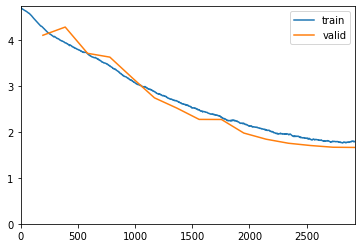Congratulations you made it to the end. You can now set any hyper-parameter value by just visualizing the validation loss for a few epochs and seeing whether our models overfit or not. The content of this notebook has been taken from the four papers by Lesli N. Smith as mentioned in the starting of the notebook and from the fastai courses taught by Jeremy Howard.

I regularly write about new research being published either or Medium or as I first tried on Jupyter notebooks. You can check my homepage where I list all of my content that I publish https://kushajveersingh.github.io/ (I am still updating the website so if the homepage is not working, just give me a few days of time).

In [ ]: